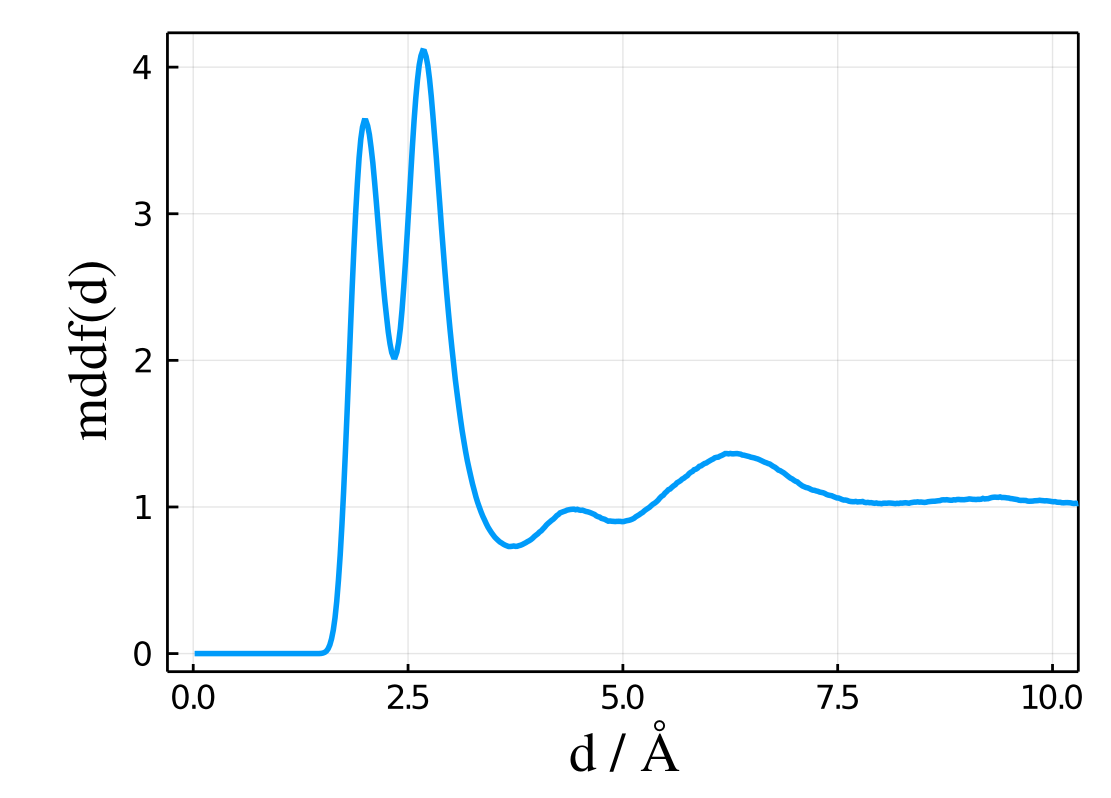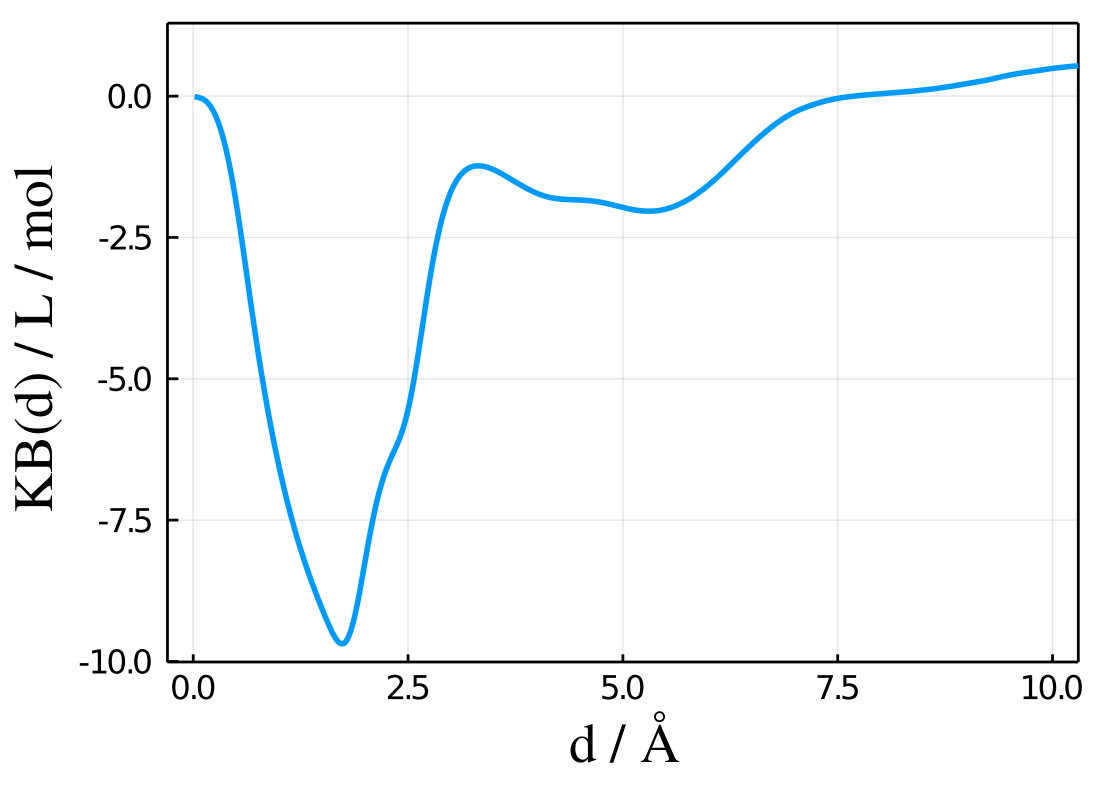# Results

The results of a MDDF calculation are returned in a data structure which contains the MDDF, KB integrals, and atomic contributions. The following section will assume that the computation was performed by calling the mddf function with

results = mddf(trajectory)

such that the results variable contain the Result data structure. By default, the histograms contain 500 bins (binstep=0.002 and cutoff=10.) such that all data-vectors will contain 500 lines.

To learn how to save and load saved data, read the next section.

## The Result data structure: main data

The most important data to be read from results are the distances, minimum-distance distribution function, and KB integrals. These data is stored in the following vectors:

## Distances of the histograms: results.d

The following vector will contain values ranging from 0. to cutoff, and the distance at each bin is the distance in that bin for which half of the volume of the bin is within d, and half of the volume is above d, if the volume was spherical:

julia> results.d
500-element Array{Float64,1}:
0.015874010519682
0.033019272488946275
⋮
9.970010030080179
9.99001000999998

## Minimum-distance distribution function: results.mddf

The results.mddf vector will contain the main result, which the minimum-distance distribution function. For a properly-sampled simulation, it will be zero at very short distances and converge to 1.0 for distances smaller than the cutoff:

julia> results.mddf
500-element Array{Float64,1}:
0.0
0.0
⋮
0.999052514965403
1.001030818286187


A typical plot of results.mddf as a function of results.d will look like:Thus, this plot was obtained with the following code:

using Plots
plot(results.d,results.mddf,xlabel="d/A",ylabel="mddf(d) / L/mol") 

## Kirkwood-Buff integral: results.kb

The results.kb vector will contain the Kirkwood-Buff integral computed as a function of the minimum-distance to the solute. For properly sampled simulations, it is expected to converge at large distances.

julia> results.kb
500-element Array{Float64,1}:
0.0
-0.3249356504752985
-2.9804719721525
⋮
0.72186381783
1.13624162115

A typical plot of results.kb as a function of results.d will look like:Thus, this plot was obtained with the following code:

using Plots
plot(results.d,results.kb,xlabel="d/A",ylabel="mddf(d) / L/mol") 

## Units

• The distance is assumed to be in Å, as this is the most common distance units in molecular simulations. The coordinates of the atoms are assumed be provided in Å.

• The minimum-distance distribution function is unit-less, since it is the ratio of the density at each distance divided by an ideal-gas density.

• The Kirkwood-Buff integrals are returned in cm³ mol⁻¹, if the coordinates were provided in Å.

Warning

If the coordinates are not in Å, the calculation will proceed normally, but the units of the KB integrals, which has units of volume per mol, should be converted to conform the length unit provided.

## Coordination number and other data

Obtaining the MDDF involves the computation of some intermediate properties that are frequently useful for additional solution structure analysis. In particular, the coordination numbers are computed. For example, the coordination number as a function from the distance to the solute can be retrieved from a Results data structure with:

coordination_number = results.coordination_number

and this data can be plotted against the distances by:

plot(result.d,results.coordination_number)

The coordination number of subgroups can also be obtained, as explained in the Coordination number section.

The complete data available is:

ParameterMeaningType of valueComment
dVector of distances of the histograms.Vector{Float64}To be used as the x coordinate on plotting any of the data.
md_countNon-normalized count of minimum distances at each d.Vector{Float64}This is the number of minimum distances found at each histogram bin, without normalization. Usually this is not interesting to analyze, because it is dependent on the bin size.
md_count_randomNumber of minimum distances found at each histogram bin for the random distribution.Vector{Float64}This is the normalization required to convert the md_count array into the minimum-distance distribution.
coordination_numberCumulative number of sites found for each histogram distance.Vector{Float64}This is the coordination number, that is, the number of sites found cumulative up to each distance, without any normalization.
coordination_number_randomCumulative site count for the random distribution.Vector{Float64}Usually not interesting for analysis.
mddfThe final distribution function.Vector{Float64}This is the MDDF computed (md_count normalized by md_count_random). It is the main result of the calculation.
kbThe final Kirkwood-Buff integral.Vector{Float64}This is the final KB integral, as a function of the integration distance from the solute. Computed as coordination_number - coordination_number_random
solute_atomAtomic contributions of the solute.Matrix{Float64}This is a matrix with nbins lines and solute.natomspermol columns, containing the atomic contributions of each solute atom to the complete MDDF.
solvent_atomAtomic contributions of the solvent.Matrix{Float64}This is a matrix with nbins lines and solvent.natomspermol columns, containing the atomic contributions of each solvent atom to the complete MDDF.
density.soluteDensity (concentration) of the solute in the complete simulation box.Float64In units of molecules/$\textrm{\AA}^3$
density.solventDensity (concentration) of the solvent in the complete simulation box.Float64In units of molecules/$\textrm{\AA}^3$
density.solvent_bulkDensity (concentration) of the solute in the bulk region.Float64In units of molecules/$\textrm{\AA}^3$
volumeVolume measures.VolumeContains the total volume of the simulation, the bulk volume, the volume of the solute domain and the shell volume of each bin of the histogram. These are computed by numerical integration from the random distributions.
filesList of files read.Vector{String}
weightsWeights of each file in the final counts.Vector{Float64}If the trajectories have different lengths or number of frames, the weights are adapted accordingly.

### Other Result parameters available which are set at Options:

ParameterMeaningType of valueComment
nbinsNumber of bins of the histograms.Int
dbulkDistance from solute of bulk solution.Float64
cutoffMaximum distance to be considered for histograms.Float64
autocorrelationThe solute is the same as the solvent?BoolAutomatically set if solute == solvent.
soluteProperties of the soluteSolSummaryContains the number of atoms, number of atoms per molecule and number of molecules of the solute.
solventProperties of the solvent.SolSummaryContains the number of atoms, number of atoms per molecule and number of molecules of the solvent.
irefatomThis is a reference atom that is used to generate random rotations and translations internally.IntCounts of the distributions for this atom are performed automatically to obtain radial (or proximal) distribution functions. Can be used for testing purposes.
rdf_countThis is the md_count minimum distance count of irefatom.Vector{Float64}This corresponds to the conventional radial distribution function if the solute contains only one atom.
rdf_count_randomMinimum distance of irefatom count for the random distribution.Vector{Float64}
rdfDistribution function computed from the irefatom distribution. It is a conventional rdf if the solvent has only one atom.Vector{Float64}
kb_rdfKirkwood-Buff integral computed from the irefatom distribution.Vector{Float64}This must converge, at long distances, to the same value as kb, and can be used for testing.
optionsCalculation options.OptionsCarries (some redundant) options set by the user.
lastframe_readLast frame read from the trajectory.Int
n_frames_readNumber of frames read from the trajectory.IntCan differ from lastframe_read if stride != 1